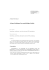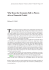# Symmetry-breaking due to Coulomb friction

## Transcription

Symmetry-breaking due to Coulomb friction
```ECCOMAS Thematic Conference on Multibody Dynamics
June 29 - July 2, 2015, Barcelona, Catalonia, Spain
Symmetry-breaking due to Coulomb friction
G. Licskó∗ , G. Csernák#
∗
Department of Applied Mechanics
Budapest University of Technology and Economics
H-1521, Budapest, Hungary
[email protected]
# MTA-BME Research Group on Dynamics of Machines and Vehicles
H-1521, Budapest, Hungary
[email protected]
Abstract
There are several mechanical systems in which the occurrence of dry (Coulomb) friction breaks the
symmetry in the structure of the corresponding equations of motion. For example, if the normal force
between two bodies depends on the acceleration of the centroids, the mass matrix may become asymmetric. This asymmetry may even lead to the loss of stability of the desired motion. The incorporation
of such effects in linear models of brakes was extensively studied, see e.g., .
The mechanical model considered in the present paper does not show this type of asymmetry in the equations of motion. However, pairs of asymmetric periodic solutions were found, with different maximal
displacements in the positive and negative directions measured from the equilibrium.
The simplest model where the existence of asymmetric solutions was pointed out was a SDoF harmonically excited linear oscillator with dry friction, with equal friction coefficients of sticking S1 and sliding
S . The dimensionless equation of motion assumes the form
ẍ + x = cos (Ω (t + t0 )) − S f (ẋ) , where

1
if ẋ > 0

[−S1 /S, S1 /S] if ẋ = 0
f (ẋ) ∈

−1
if ẋ < 0
(1)
is a set-valued function. The symmetry-breaking solutions were derived analytically for the case of
equal coefficients of friction (S1 = S) and it was shown that they exist only at certain sub-harmonic
resonant frequencies Ω = 1/2, 1/4, 1/6, etc. Later it was found that the frequency domain of asymmetric
solutions opens up if the ratio of sticking and sliding coefficients of friction is large, see Figure 1.
Figure 1: An asymmetric periodic solution at Ω = 0.49, S = 0.2 and S1 = 0.4.
The special non-smooth bifurcations of so-called Filippov systems have already been classified and several methods were developed for their analysis . However, the analytical treatment of the case S1 6= S
is rather difficult, because the resulting non-smooth equation of motion does not belong to the group of
Filippov systems. In the examined case the vector field corresponding to sticking cannot be expressed
as a convex combination of the vector fields that govern the sliding with positive or negative velocities.
Another field of mathematics that deals with dry friction systems uses the notion of maximal monotone
operators  – but the difference of sticking and sliding coefficient of friction means that the corresponding operator is not maximal monotone.
This is why we opted for a numerical search for special solutions. We used a brute-force approach and
a continuation method, too. The obtained bifurcation diagram, shown in Figure 2, revealed the existence
of chaotic solutions that are related to the symmetry-breaking. The parameter domains of periodic,
xstick
1.5
1
0.5
0
0.1
S/S1
0.2
Figure 2: The positions of sticking segments versus the ratio S/S1 . Ω = 0.5, S1 = 0.4.
chaotic and transient chaotic solutions were explored and the Lyapunov exponent of the system was also
calculated at several parameter values .
The aforementioned symmetry-breaking is related to a (non-smooth) crossing-sliding bifurcation. However, traces of symmetry-breaking behaviour were detected in a similar model with smooth LuGre friction, as well. Thus, we expect to encounter such special motions in real mechanical systems, too. The
construction of a test rig is under way to proove the computational results. The different coefficients of
friction will be achieved with the help of spherical roller thrust bearings where the starting torque and
operational resistance torque are different.
Acknowledgements
This research was supported by the Hungarian National Science Foundation under grant no. OTKA K
83890. The research leading to these results has received funding from the European Research Council
under the European Union’s Seventh Framework Programme (FP/2007-2013) / ERC Grant Agreement
n. 340889.
References

D. Hochlenert, G. Spelsberg-Korspeter, P. Hagedorn. A note on safety-relevant vibrations induced
by brake squeal. Journal of Sound and Vibration, Vol. 329, pp. 3867–3872, 2010.

G. Csernák, G. Stépán and S. W. Shaw. Sub-harmonic resonant solutions of a harmonically excited
dry friction oscillator. Nonlinear Dynamics, Vol. 50, No. 1-2, pp. 93–109, 2007.

M. Bernardo, C. Budd, A.R. Champneys, P. Kowalczyk. Piecewise-smooth Dynamical Systems:
Theory and Applications. Springer, Series: Applied Mathematical Sciences, Vol. 163, 2008.

R. Leine, H. Nijmeijer. Dynamics and Bifurcations of Non-Smooth Mechanical Systems.
Springer, Lecture Notes in Applied and Computational Mechanics Vol. 18, 2004.

G. Licsko, G. Csernak. On the chaotic behaviour of a simple dry-friction oscillator. Mathematics
and Computers in Simulation, Vol. 95, pp. 55–62, 2014.
```

### PROFILES PROJECT AMBROSI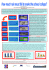### HabaCHAIN TS Material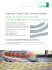### Simple Friction Experiments - Veletrh nápadů učitelů fyziky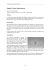### Tribotester - ASEC Kunststoffen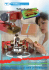### Johns Hopkins University Tristan A. Sharp, Lars Pastewka, Mark O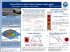### Rock Shoes Tech Sheet 2011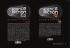### μ - METER FRICTION MEASUREMENT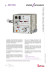### Solutions to Sample Problems 1.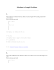### Equipments and Materials for Tribotesting in Open Space on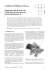### diaaf short sellers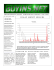### Preload (mN) - Nano Ontario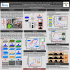### PHY131H1F - Class 9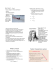### nwbo short sellers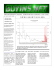### Document 6575243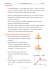### Longfellow pdf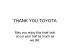### Static: F ` Âµ F Kinetic F ` Âµ F Metal on metal 0.15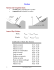### 1. What is unit vector? 2. State the principle of transmissibility.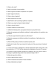### Inter-particle force and stress models for wet and dry particulate flow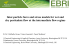### MOTORUP XTREME 800 Engine Treatment PDF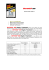### Advanced techniques for nanotribological studies